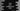# Java program to print multiplication table of a number## Java program to print the Multiplication table of a number :

We can use one for loop, while loop or do-while loop to print a multiplication table for any number. Using Scanner, we will get the number for which the table should be printed :

### Example program :

``````import java.util.Scanner;

public class Main {

public static void main(String[] args) {
Scanner sc = new Scanner(System.in);
int no = 0;

System.out.println("Enter the number : ");
no = sc.nextInt();

for (int i = 0; i < 10; i++) {
System.out.println(no + " * " + (i + 1) + " = " + (no * (i + 1)));
}

}

}``````

### Example :

``````Enter the number :
9
9 * 1 = 9
9 * 2 = 18
9 * 3 = 27
9 * 4 = 36
9 * 5 = 45
9 * 6 = 54
9 * 7 = 63
9 * 8 = 72
9 * 9 = 81
9 * 10 = 90``````